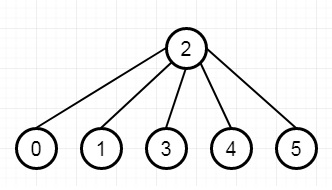# Time Needed to Inform All Employees in C++

C++Server Side ProgrammingProgramming

Suppose we have a company has n employees with a unique ID for each employee. These IDs are ranging from 0 to n - 1. The head of the company has is the one with headID. Each employee has one direct manager given in the manager array where manager[i] is the direct manager of the i-th employee, manager[headID] = -1. Also it's guaranteed that the subordination relationships have a tree-like structure. Here the head of the company wants to inform all the employees of the company of an urgent piece of news. He can inform his direct subordinates and they will inform their subordinates and so on until all employees know about the urgent news. The i-th employee needs the informTime[i] minutes to inform all of his direct subordinates (So after informTime[i] minutes, all his direct subordinates can start spreading the news). We have to find the number of minutes needed to inform all the employees about the urgent news. So if the input is like n = 6, headID = 2, manager = [2,2,-1,2,2,2], infromTime = [0,0,1,0,0,0], then the output will be 1.To solve this, we will follow these steps −

• ret := 0

• define an array called the graph of size n, set root := -1

• for i in range 0 to the size of manager array

• u := managet[i] and v := i

• if u is -1, then set root := v, and go for next iteration

• insert v into graph[u]

• define a queue q, insert root into q, and define an array called time, of size n

• until the q is not empty

• curr := front element of q, and delete front element from q

• if size of the list of graph[curr] is 0, then skip to the next iteration

• for i in range 0 to the size of the list of graph[curr]

• insert graph[curr, i] into q

• time[graph[curr, i]] := time[curr] + informTime[curr]

• for i in range 0 to n – 1: ret := max of ret and time[i]

• return ret

## Example (C++)

Let us see the following implementation to get a better understanding −

Live Demo

#include <bits/stdc++.h>
using namespace std;
class Solution {
public:
int numOfMinutes(int n, int headID, vector<int>& manager, vector<int>& informTime) {
int ret = 0;
vector <int> graph[n];
int root = -1;
for(int i = 0; i < manager.size(); i++){
int u = manager[i];
int v = i;
if(u == -1) {
root = v;
continue;
}
graph[u].push_back(v);
}
queue <int> q;
q.push(root);
vector <int> time(n);
while(!q.empty()){
int curr = q.front();
q.pop();
if(!graph[curr].size()) continue;
for(int i = 0; i < graph[curr].size(); i++){
q.push(graph[curr][i]);
time[graph[curr][i]] = time[curr] + informTime[curr];
}
}
for(int i = 0; i <n; i++)ret = max(ret, time[i]);
return ret;
}
};
main(){
vector<int> v = {2,2,-1,2,2,2}, v1 = {0,0,1,0,0,0};
Solution ob;
cout << (ob.numOfMinutes(6, 2, v, v1));
}

## Input

6
2
[2,2,-1,2,2,2]
[0,0,1,0,0,0]

## Output

1